Geometric Progression(GP): Formulae based

Chapter 8 Class 11 Sequences and Series
Concept wise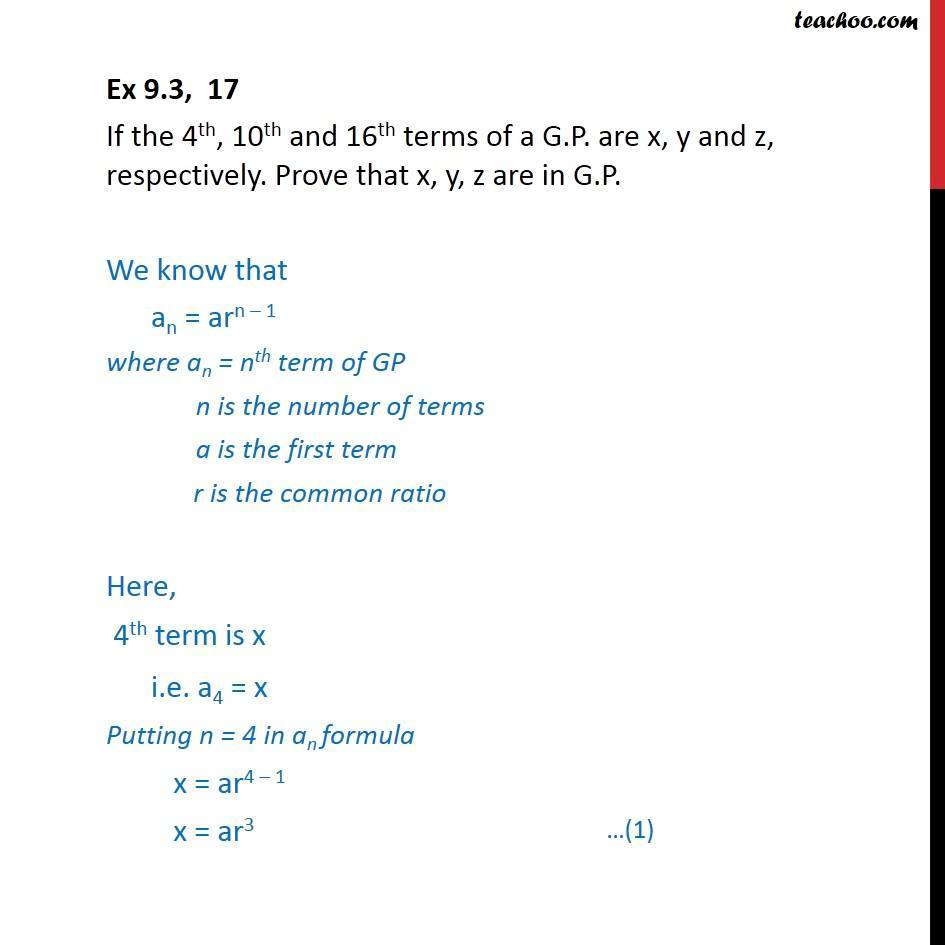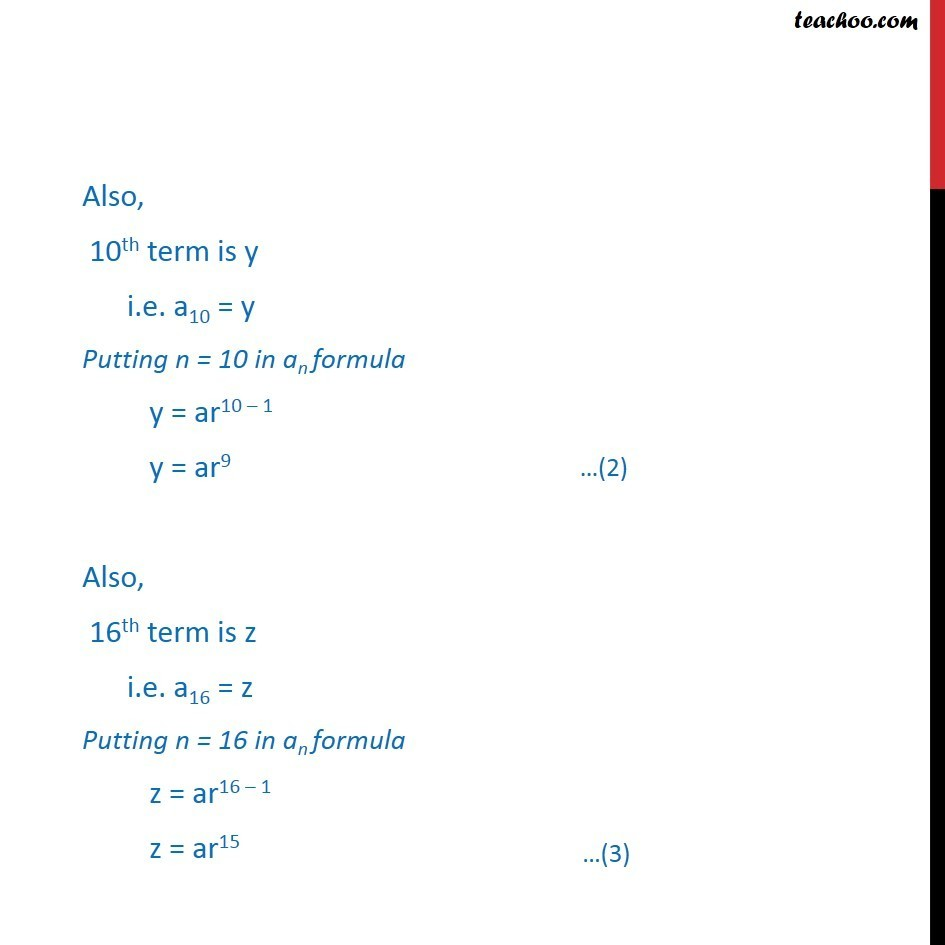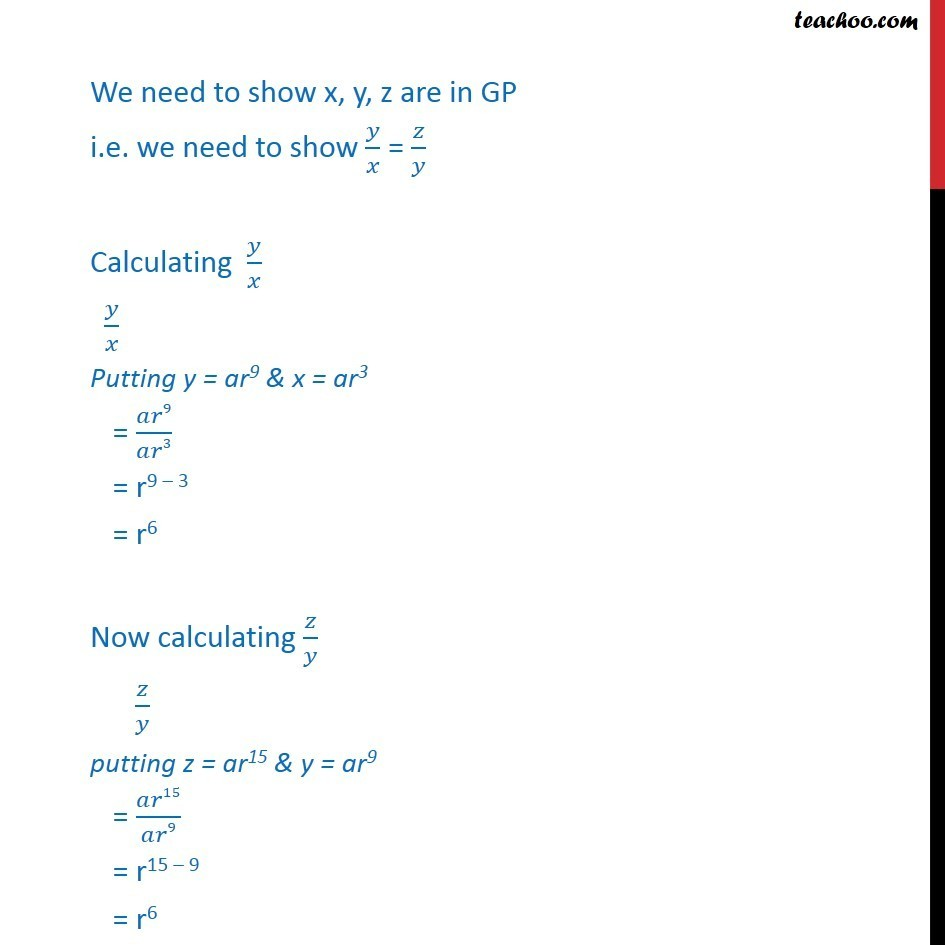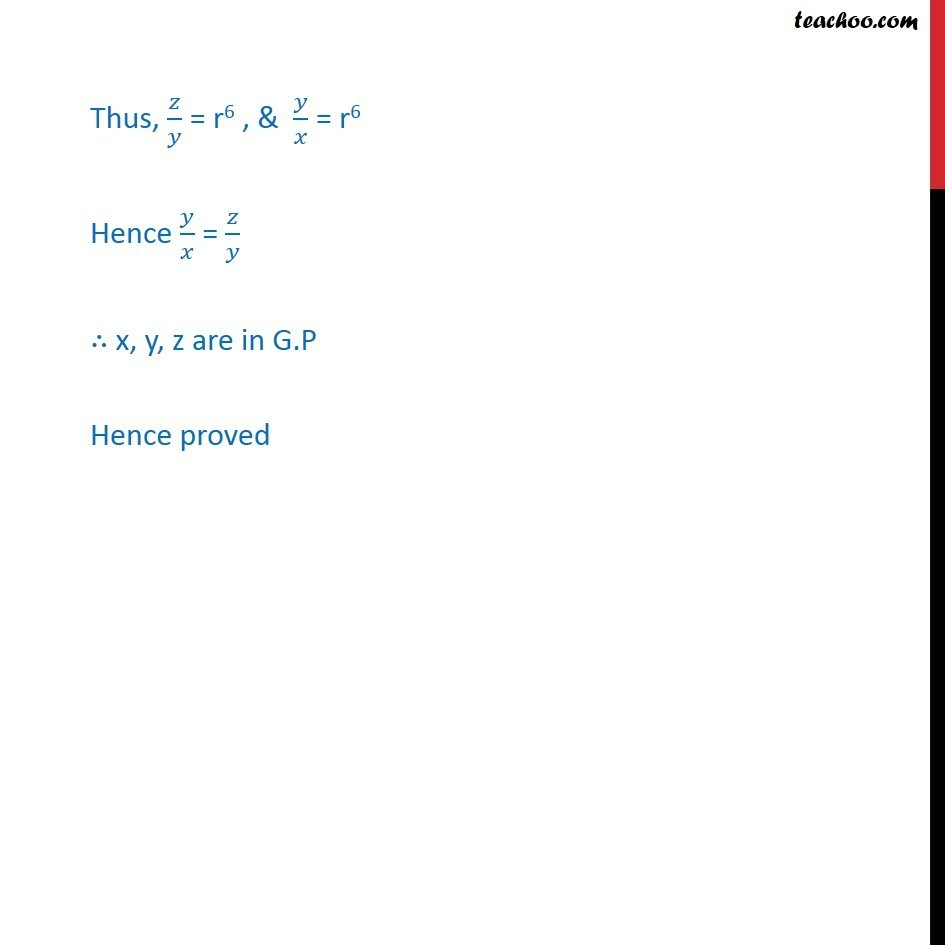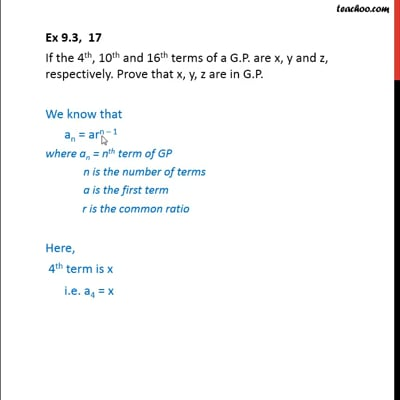This video is only available for Teachoo black users

Learn in your speed, with individual attention - Teachoo Maths 1-on-1 Class

### Transcript

Ex 8.2, 17 If the 4th, 10th and 16th terms of a G.P. are x, y and z, respectively. Prove that x, y, z are in G.P. We know that an = arn 1 where an = nth term of GP n is the number of terms a is the first term r is the common ratio Here, 4th term is x i.e. a4 = x Putting n = 4 in an formula x = ar4 1 x = ar3 Also, 10th term is y i.e. a10 = y Putting n = 10 in an formula y = ar10 1 y = ar9 Also, 16th term is z i.e. a16 = z Putting n = 16 in an formula z = ar16 1 z = ar15 We need to show x, y, z are in GP i.e. we need to show / = / Calculating / / Putting y = ar9 & x = ar3 = 9/ 3 = r9 3 = r6 Now calculating / / putting z = ar15 & y = ar9 = 15/ 9 = r15 9 = r6 Thus, / = r6 , & / = r6 Hence / = / x, y, z are in G.P Hence proved